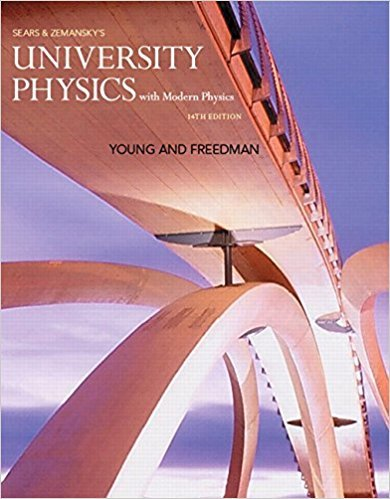×
×

# An object is moving along the x-axis. At t = 0 it hasISBN: 9780321973610 228

## Solution for problem 2.81 Chapter 2

University Physics with Modern Physics (1) | 14th Edition

• Textbook Solutions
• 2901 Step-by-step solutions solved by professors and subject experts
• Get 24/7 help from StudySoup virtual teaching assistantsUniversity Physics with Modern Physics (1) | 14th Edition

4 5 1 259 Reviews
20
3
Problem 2.81

An object is moving along the x-axis. At t = 0 it has velocity v0x = 20.0 m>s. Starting at time t = 0 it has acceleration ax = -Ct, where C has units of m>s 3 . (a) What is the value of C if the object stops in 8.00 s after t = 0? (b) For the value of C calculated in part (a), how far does the object travel during the 8.00 s?

Step-by-Step Solution:
Step 1 of 3
Step 2 of 3

Step 3 of 3

##### ISBN: 9780321973610

Unlock Textbook Solution